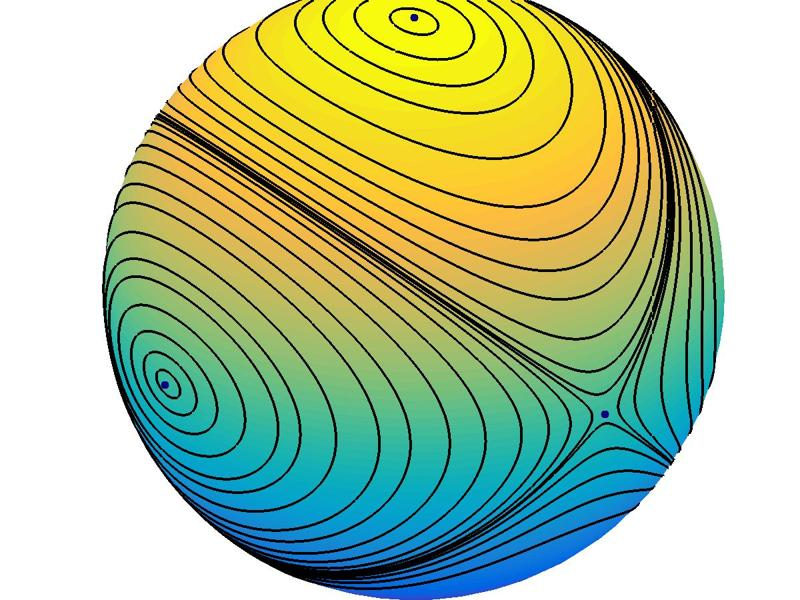# Tumbling Box ODE

A rectangular box, such as a book or a cell phone, thrown in the air can tumble stably about its longest axis, or about its shortest axis, but not about its middle axis.

### Contents

#### Angular Momenta

In his latest book, Differential Equations and Linear Algebra, my colleague Gilbert Strang describes an interesting model problem made famous among MIT students by another MIT professor, Alar Toomre. If you throw a rectangular box in the air with a twist, you can make it tumble stably about its longest axis, or about its shortest axis. But, if the lengths of the three sides of the box are different, you cannot make it tumble about its middle-sized axis.

To describe the dynamics of the box, discount the effects of gravity by using a coordinate system centered in the box and that moves with it. Let $x(t)$, $y(t)$, and $z(t)$ be the angular momenta about the three principal axes, and let $I_1$, $I_2$, and $I_3$ be the moments of inertia about these axes. Then Euler's equations are

$${ d \over dt} \left( \begin{array}{c} x \\ y \\ z \end{array} \right) = \left( \begin{array}{r} ayz \\ bxz\\ cxy \end{array} \right)$$

where

$$a = 1/I_3 - 1/I_2, \ \ b = 1/I_1 - 1/I_3, \ \ c = 1/I_2 - 1/I_1$$

If we have

$$I_1 = 1, \ \ I_2 = 1/2, \ \ I_3 = 1/3$$

the equations become simply

$${ d \over dt} \left( \begin{array}{c} x \\ y \\ z \end{array} \right) = \left( \begin{array}{r} yz \\ -2xz\\ xy \end{array} \right)$$

#### Critical points

There are six critical points. Any solution to the Euler equations that starts exactly at one of these points remains there.

$$X = \pm \left( \begin{array}{c} 1 \\ 0 \\ 0 \end{array} \right), \ \ Y = \pm \left( \begin{array}{c} 0 \\ 1 \\ 0 \end{array} \right), \ \ Z = \pm \left( \begin{array}{c} 0 \\ 0 \\ 1 \end{array} \right)$$.

It turns out that any solution that starts out with $x^2+y^2+z^2=1$ retains that property, so solutions travel on the surface of the unit sphere. If you think of the sphere as the earth with $+Z$ at the North Pole, then $+X$ is the point where the Greenwich meridian crosses the equator. This is in the eastern Atlantic, off the coast of West Africa. $+Y$ is the point where the $90^\circ$ meridian east crosses the equator. This is in Indian Ocean, west of Sumatra.

#### Stable critical points

To see what happens near a critical point, we need the Jacobian.

$$J = \left[ \begin{array}{rrr} 0 & z & y \\ -2z & 0 & -2x \\ y & x & 0 \end{array} \right]$$

At $+X$

$$J = \left[ \begin{array}{rrr} 0 & 0 & 0 \\ 0 & 0 & -2 \\ 0 & 1 & 0 \end{array} \right]$$

The eigenvalues of $J$ are pure imaginary, namely $\lambda = \pm \sqrt{2}i$, along with $\lambda = 0$. So near $+X$ the solutions behave locally like $\cos{(\sqrt{2}t)}$ and $\sin{(\sqrt{2}t)}$. This critical point, which corresponds to rotation about the long axis, is a stable center. The critical point $-X$, which corresponds to rotation about the long axis in the opposite direction, is also a stable center.

At $+Z$

$$J = \left[ \begin{array}{rrr} 0 & 1 & 0 \\ -2 & 0 & 0 \\ 0 & 0 & 0 \end{array} \right]$$

We have the same eigenvalues and so rotation about the short axis is also a stable center.

#### Unstable critical points

The middle axis, $+Y$, is a different story. Here

$$J = \left[ \begin{array}{rrr} 0 & 0 & 1 \\ 0 & 0 & 0 \\ 1 & 0 & 0 \end{array} \right]$$

The eigenvalues of $J$ are real, namely $\lambda = 1, 0,$ and $-1$. Near $+Y$ and $-Y$ the solutions have components that behave locally like $e^t$. These are unstable saddle points.

The surface of the sphere can be divided into four quadrants, with one of the stable critical points, $\pm X$ or $\pm Z$, at the center of each quadrant. Any solution to the differential equation is periodic and describes an orbit that remains within one of the quadrants, circulating around its center.

If the initial point is near the center of a quadrant, the orbit is nearly elliptical and the orbit has period close to $\sqrt{2}\pi$. If the initial point is near the boundary of a quadrant, the orbit sticks near that boundary, making sharp turns at the two saddle points $\pm Y$. The period increases as the initial point gets further from the center.

I think of these as giant atmospheric currents that circulate around one-fourth of a planet.

#### Tumbling_box.m

Here is a link to a program, tumbling_box.m, that uses ode45 to solve these equations and produce the following graphic. I invite you to download the program and experiment yourself. You are presented with a sphere that is empty except for dots at three critical points. You can click repeatedly to provide the initial conditions for the orbits.

You can also click on icons in the figure window toolbar to zoom in and out, and rotate and pan over the sphere. If you zoom in to the stable centers points, you can see the elliptical orbits associated with stability. If you zoom in to the saddle points, you can see the hyperbolic orbits associated with instability. If you rotate the sphere, you can follow one of the unstable orbits around the sphere, back to its starting point.

Tumbling_box includes ode45 event handling code to determine the length of a period of the solution. It also has mouse button down code to interpret the coordinates of a mouse click as a point on the surface of the unit sphere.#### Reference

Gilbert Strang, Differential Equations and Linear Algebra, Wellesley-Cambridge Press, 2014, 502pp. <http://www.wellesleycambridge.com>

Published with MATLAB® R2015a

|